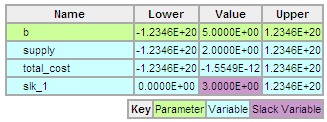## Slack Variable Tutorial

Slack variables are defined to transform an inequality expression into an equality expression with an added slack variable. The slack variable is defined by setting a lower bound of zero (>0).

 Inequality Constraint Form x > b Equality Constraint Form with Slack Variable x = b + slack slack > 0

### Example Problem

\begin{align}\min \quad & cost_{total}\\\mathrm{subject\;to} \quad & supply<b\\& cost_{total}=(supply-2)^2\end{align}

Gekko (Python) Solution

# Solve slack variable problem
#  Minimize   total_cost
#  Subject to supply < b
from gekko import GEKKO

b = 5
m = GEKKO(remote=False)
supply = m.Var()
total_cost = m.Var()

m.Equation(supply<b)
m.Equation(total_cost==(supply-2)**2)
m.Minimize(total_cost)

m.solve()

print(supply.value)
print(total_cost.value)

APMonitor SolutionClick to Solve Slack Variable Optimization Problem Online### Inequality Constraints

In Gekko Optimization Suite and the APMonitor Modeling Language, inequality constraints are automatically translated into equality constraints with slack variables. In APMonitor, slack variables can also be defined by starting a variable name with slk. When the model is parsed at run-time, any variable beginning with slk is automatically assigned a lower value of zero. Alternatively, inequality constraints are automatically converted to equality constraints with a slack variable.